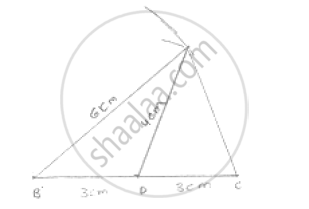# Construct a Triangle Abc Such that Bc = 6 Cm, Ab = 6 Cm and Median Ad = 4 Cm. - Mathematics

Construct a triangle ABC such that BC = 6 cm, AB = 6 cm and median AD = 4 cm.

#### SolutionSteps of construction:
Step 1: Draw a line segment BC of 6cm.
Step 2: Take midpoint D of BC.
Step 3: with center B and D and radii 6cm and 4cm draw two arcs which intersects each other A
Step 4: Join AB, AD and AC
∴ Δ ABC is the required triangle

Concept: Some Constructions of Triangles
Is there an error in this question or solution?

#### APPEARS IN

RD Sharma Mathematics for Class 9
Chapter 16 Constructions
Exercise 16.3 | Q 6 | Page 18

Share